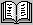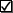DefinitionsMonotonic functions are functions that tend to move in only one direction as x increases. A monotonic increasing function always increases as x increases, i.e. f(a)>f(b) for all a>b. A monotonic decreasing function always decreases as x increases, i.e. f(a)<f(b) for all a<b.One-to-one correspondence is so fundamental to counting that we don't even think about it. When we count out a deck of cards, we say, 1, 2, 3, ... , 52, and as we say each number we lay down a card. Each number corresponds to a card. Technically, we can say that we have put the cards in the deck and the numbers from 1 to 52 in a one-to-one correspondence with each other. If we count out a second deck and in the same way and count to exactly 52 when we lay out the cards, , we know that both decks have the same number of cards. This means that we could pair up the cards, one from each deck, and not have any cards left over when all the pairs had been made. By putting the cards in each deck in a one-to-one correspondence with each other, we are sure that both decks have the same number of cards. These things are so obvious they seem silly. However, if we want to know the size of an unknown quantity, but the counting task is tricky, we can try to put the unknown quantity in one-to-one correspondence with some known quantity. This is the strategy that Georg Cantor used to compare different sizes of infinity.

This definition was taken from http://www.c3.lanl.gov/mega-math/gloss/Bayes rule:Forward dynamic programming: In forward dynamic programming each time instant k has to be processed completely before going to the time instant k+1. After that it suffices that each state holds only the most likely path from the initial state to that state and the probability of this path. This is again due to the property of the first-order Markov process.